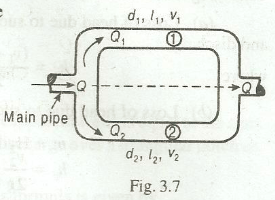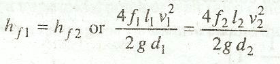Pipes in Parallel

How do Pipes in Parallel work from fluid mechanics' point of view? What is rate of discharge in main pipe and loss of head in each pipe?

Figure below shows a system of pipes in parallel. In such a case(a) The rate of discharge in the main pipe is equal to the sum of discharges in each of the parallel pipes, i.e.

Q =Q1 + Q2,

(b) The loss of head in each pipe is same, i.e.